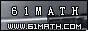Today is . WELCOME: huohai3983/44663  Home | Reg Login | All user | setHomePage | BookmarkHome | Math Test Online | Addition | Subtraction | Multiplication | Division | Time | Worksheet(JPG/PDF) | CAI | Flash Games |Recommendation articleArticle searchHome--maths--First Grade--Adding Tens

How to add two-digit numbers (for example 40 + 50).

• Place one number above the other so the tens' place digits and ones' place digits are lined up. Draw a line under the bottom number.
```40
50
```
• Add the two ones' place digits. (0 + 0 = 0). Place the zero below the line in the ones' place column.
```40
50
0
```
• Add the numbers in the tens' place column (4 + 5 = 9) and place the answer below the line and to the left of the ones' place sum
```40
50
90```

#### Find the Sum of the two Numbers.

 =

2006-10-02 20:05:47
Viewed 2108 times CloseGreatmathsites

### Translate

 Thank you to online math channels' Mathematics Department for hosting these lessons. Copyright © 2006 - 2008 GZtrain Inc. All Rights Reserved